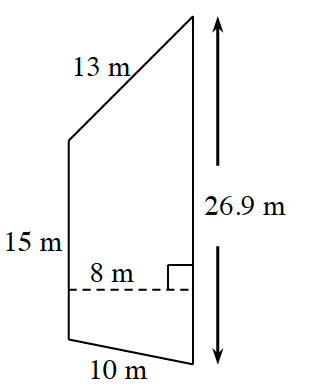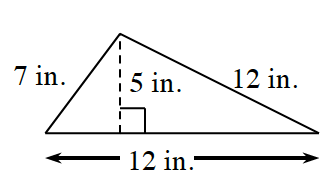### Home > CC1MN > Chapter 9 > Lesson 9.2.1 > Problem9-47

9-47.

Find the area and perimeter of each figure below.

1.Use the formula below to find the area of a trapezoid:

$\text{Area}=(\text{height})\left ( \frac{\text{base}_{1}+\text{base}_{2}}{2} \right )$

Which two sides are parallel? These are the bases.
Use their dimensions in the formula given above.

$\text{Area}=(\text{8 m}) \! \left ( \frac{\text{15 m + 26.9 m}}{2} \right )\\ =167.6 \text{ m}^{2}$

1.Use the formula below to find the area of a triangle:

$\text{Area}=\frac{1}{2}(\text{base})(\text{height})$